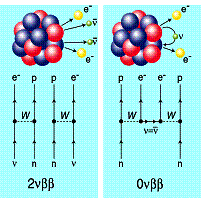# Double Beta-decayNeutrino oscillations have been observed in experiments with solar as well as atmospheric neutrinos. As a result, we know that neutrinos are not massless as described by the standard model of particle physics, but have a finite rest mass. However, oscillation experiments are sensitive to mass differences between different neutrino flavours only, which have been found to be in the order of 50 meV. In order to derive an absolute neutrino mass, experiments on the nuclear double beta decay are carried out.

The nuclear double beta decay is an extremely rare radioactive decay mode where an isotope changes its charge by two units while its number of nucleons is preserved.

Two reaction modes are at the center of attention, the neutrinoless and the two-neutrino decay. The latter emits two electrons and two neutrinos. It is allowed by the standard model and has even been directly observed in a number of cases. Typically, half lives are in the order of 1019 years (compare to the age of our universe which is about < 2x1010 years). The second mode, in which no neutrino is emitted, is even more interesting. Here the conservation of lepton number, a central postulation of the standard model, is violated. Namely, electrons may not simply appear from nowhere, but for each created electron (lepton number 1) an anti-neutrino (lepton number -1) is needed to agree the balance.

The neutrinoless decay mode is usually described as two sequential ordinary beta decays. The first one emits a neutrino, which is immediately re-absorbed by the second decay. This is however only possible if neutrino and anti-neutrino are identical particles, which in turn is allowed by the theory of weak interaction if the neutrino has a rest mass!

Indeed, theorists have shown that the neutrinoless double beta decay half life of a nucleus is directly connected with the neutrino mass. In other words, a measurement of the half life would give us the neutrino mass! As such half lives are expected to be extremely long (about 1028 years!), measurements are a demanding challenge which has not been mastered yet.

At this point, one has to think about a further factor: the nuclear structure of the involved isotopes. These so-called nuclear matrix elements are computed by sophisticated model calculations. Some important matrix elements are also accessible through experiments. A calibration of the model calculations on the basis of such data is absolutely essential in order to obtain, in case of the discovery of neutrinoless double beta decay, a precise neutrino mass out of the eventually observed half life. Suchlike measurements are carried out by our group at the accelerator insttute KVI in Groningen, where a setup was installed which produces data with a worldwide unrivaled, unprecedented precision.